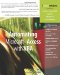# Introducing Array Variables

 < Day Day Up >

 So far, the variables you've encountered have been straightforward in that all variables have a purpose, a data type, and eventually, a value. Sometimes variables aren't quite so simple. Sometimes a variable can equal more than one value at the same time. In this chapter, you learn how array variables store more than one value. In general terms, an array refers to a large group of similar items. In mathematical terms, an array is rectangular set of values in rows and columns. A VBA array is a set of fixed values, called elements, that share the same data type and name. VBA interprets and processes the group of values as one variable. Array variables are useful anytime you have a set of related values that you need to store. For instance, if you loop through the same code, grabbing values and storing them in a temporary table, you might consider an array to store those values instead. Temporary tables require additional code to create, maintain, and then destroy. Whatever your reason, anytime you find yourself thinking in terms of storing a set of related values, consider using an array.
 < Day Day Up >Automating Microsoft Access with VBA
ISBN: 0789732440
EAN: 2147483647
Year: 2003
Pages: 186